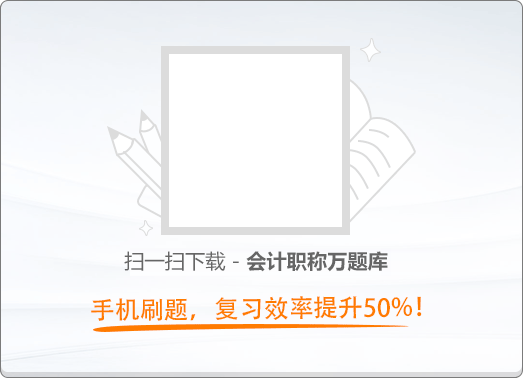120:002011年初级会计实务真题

1
(单项选择题)

• A.

收回应收款项

• B.

计提资产减值准备

• C.

盈余公积转增资本

• D.

接受股东追加投资

• A
• B
• C
• D

2
(单项选择题)

• A.

7 700

• B.

8 700

• C.

9 200

• D.

10 700

• A
• B
• C
• D

3
(单项选择题)

• A.

预计产品质量保证损失

• B.

聘请中介机构年报审计费

• C.

专设售后服务网点的职工薪酬

• D.

企业负担的生产职工养老保险费

• A
• B
• C
• D

4
(单项选择题)

2010年5月，甲公司销售商品实际应交增值税38万元，应交消费税20万元，提供运输劳务实际应交营业税15万元；适用的城市维护建设税税率为7%，教育费附加为3%。假定不考虑其他因素，甲公司当月应列入利润表“营业税金及附加”项目的金额为（　）万元。

• A.

7.3

• B.

38.5

• C.

25.8

• D.

80.3

• A
• B
• C
• D

5
(单项选择题)

• A.

收到的财政贴息

• B.

收到先征后返的增值税

• C.

收到政府作为资本性投入的财政拨款

• D.

收到政府为支持企业技术创新无偿拨款付的款项

• A
• B
• C
• D

6
(单项选择题)

• A.

普通年金

• B.

即付年金

• C.

递延年金

• D.

永续年金

• A
• B
• C
• D

7
(单项选择题)

• A.

固定资产报废净损失

• B.

支付的矿产资源补偿费

• C.

预计产品质量保证损失

• D.

基本生产车间设备计提的折旧费

• A
• B
• C
• D

8
(单项选择题)

2010年1月1日，甲公司采用分期付款方式购入大型设备一套，当日投入使用。合同约定的价款为2 700万元，分3年等额支付；该分期支付购买价款的现值为2 430万元。假定不考虑其他因素，甲公司该设备的入帐价值为（　）万元。

• A.

810

• B.

2 430

• C.

900

• D.

2 700

• A
• B
• C
• D

9
(单项选择题)

• A.

借记“应收帐款”科目 贷记“银行存款”科目

• B.

借记“其他货币资金”科目 贷记“银行存款”科目

• C.

借记“其他应收款”科目 贷记“银行存款”科目

• D.

借记“材料采购”科目 贷记“其他货币资金”科目

• A
• B
• C
• D

10
(单项选择题)

• A.

2 400

• B.

3 200

• C.

6 000

• D.

20 000

• A
• B
• C
• D

11
(单项选择题)

• A.

250

• B.

292.5

• C.

286

• D.

328.5

• A
• B
• C
• D

12
(单项选择题)

• A.

事业结余

• B.

固定基金

• C.

专用基金

• D.

应缴预算款

• A
• B
• C
• D

13
(单项选择题)

• A.

收到的现金股利

• B.

支付的银行借款利息

• C.

收到的设备处置价款

• D.

支付的经营租赁租金

• A
• B
• C
• D

14
(单项选择题)

• A.

500

• B.

1 125

• C.

625

• D.

3 375

• A
• B
• C
• D

15
(单项选择题)

• A.

定额比例法

• B.

不计算在产品成本法

• C.

约当产量比例法

• D.

在产品按固定成本计算法

• A
• B
• C
• D

16
(单项选择题)

2010年1月1日，甲公司以1 600万元购入乙公司30%的股份，另支付相关费用8万元，采用权益法核算。取得投资时，乙公司所有者权益的账面价值为5 000万元（与可辨认净资产的公允价值相同）。乙公司2010年度实现净利润300万元。假定不考虑其他因素，甲公司该长期股权投资2010年12月31日的账面余额为（　）万元。

• A.

1 590

• B.

1 598

• C.

1 608

• D.

1 698

• A
• B
• C
• D

17
(单项选择题)

• A.

一般纳税企业进口原材料支付的关税

• B.

一般纳税企业购进原材料支付的增值税

• C.

小规模纳税企业购进原材料支付的增值税

• D.

一般纳税企业进口应税消费品支付的消费税

• A
• B
• C
• D

18
(单项选择题)

2009年12月31日，甲公司购入一台设备并投入使用，其成本为25万元，预计使用年限5年，预计净残值1万元，采用双倍余额递减法计提折旧。假定不考虑其他因素，2010年度该设备应计提的折旧为（　）万元。

• A.

4.8

• B.

8

• C.

9.6

• D.

10

• A
• B
• C
• D

19
(单项选择题)

• A.

应付租入包装物租金

• B.

应付融资租入固定资产租金

• C.

结转到期无力支付的应付票据

• D.

应付由企业负担的职工社会保险费

• A
• B
• C
• D

21
(单项选择题)

• A.

18 500

• B.

18 600

• C.

19 000

• D.

20 000

• A
• B
• C
• D

22
(单项选择题)

• A.

“事业结余”科目借方余额

• B.

“事业结余”科目贷方余额

• C.

“经营结余”科目借方余额

• D.

“经营结余”科目贷方余额

• A
• B
• C
• D

23
(单项选择题)

• A.

资本溢价

• B.

任意盈余公积

• C.

未分配利润

• D.

法定盈余公积

• A
• B
• C
• D

24
(单项选择题)

• A.

12%

• B.

16%

• C.

16.2%

• D.

30%

• A
• B
• C
• D

25
(单项选择题)

• A.

200

• B.

-200

• C.

500

• D.

-500

• A
• B
• C
• D